### 2. TUNNEL VISION: THE INFLATIONARY SOLUTION

Inflation can be described simply as any period of the Universe's evolution in which the size of the Universe is accelerating. This surprisingly simple type of expansion leads to our observed universe without invoking special initial conditions. The active ingredient of the inflationary remedy to the structure, horizon and flatness problems is rapid exponential expansion sometime within the first picosecond ( = trillionth of a second = 10-12 s) after the big bang. If the structure, flatness and horizon problems are so easily solved, it is important to understand how this quick cure works. It is important to understand the details of expansion and cosmic horizons. Also, since our Universe is becoming more-dominated every day (Fig. 3), we need to prepare for the future. Our descendants will, of necessity, become more and more familiar with inflation, whether they like it or not. Our Universe is surrounded by inflation at both ends of time.

The general relativistic description of an homogeneous, isotropic universe is based upon the Friedmann-Robertson-Walker (FRW) metric for which the spacetime interval ds, between two events, is given by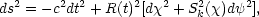(1)

where c is the speed of light, dt is the time separation, d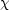is the comoving coordinate separation and d2 = d2 + sin2d2, whereandare the polar and azimuthal angles in spherical coordinates. The scale factor R has dimensions of distance. The function Sk() = sin,or sinhfor closed (positive k), flat (k = 0) or open (negative k) universes respectively (see e.g. Peacock 1999 p. 69).

In an expanding universe, the proper distance D between an observer at the origin and a distant galaxy is defined to be along a surface of constant time (dt = 0). We are interested in the radial distance so d= 0. The FRW metric then reduces to ds = Rdwhich, upon integration, becomes,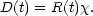(2)

Taking the time derivative and assuming that we are dealing with a comoving galaxy (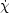= 0) we have,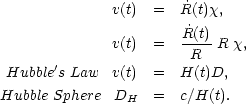(3) (4) (5) (6)

The Hubble sphere is the distance at which the recession velocity v is equal to the speed of light. Photons have a peculiar velocity of c =R, or equivalently photons move through comoving space with a velocity= c / R. The comoving distance traveled by a photon is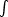dt, which we can use to define the comoving coordinates of some fundamental concepts: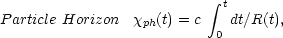(7)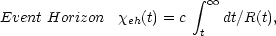(8)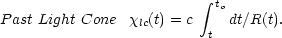(9)

Only the limits of the integrals are different. The horizons, cones and spheres of Eqs. 6 - 9 are plotted in Fig. 1.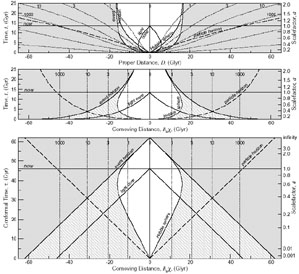Figure 1. Expansion of the Universe. We live on the central vertical worldline. The dotted lines are the worldlines of galaxies being expanded away from us as the Universe expands. They are labeled by the redshift of their light that is reaching us today, at the apex of our past light cone. Top: In the immediate past our past light cone is shaped like a cone. But as we follow it further into the past it curves in and makes a teardrop shape. This is a fundamental feature of the expanding universe; the furthest light that we can see now was receding from us for the first few billion years of its voyage. The Hubble sphere, particle horizon, event horizon and past light cone are also shown (Eqs. 6 - 9). Middle: We remove the expansion of the Universe from the top panel by plotting comoving distance on the x axis rather than proper distance. Our teardrop-shaped light cone then becomes a flattened cone and the constant proper distance of the event horizon becomes a shrinking comoving event horizon - the active ingredient of inflation (Section 2.2). Bottom: the radius of the current observable Universe (the particle horizon) is 47 billion light years (Glyr), i.e., the most distant galaxies that we can see on our past light cone are now 47 billion light years away. The top panel is long and skinny because the Universe is that way - the Universe is larger than it is old - the particle horizon is 47 Glyr while the age is only 13.5 Gyr - thus producing the 3 : 1(47 : 13.5) aspect ratio. In the bottom panel, space and time are on the same footing in conformal/comoving coordinates and this produces the 1 : 1 aspect ratio. For details see Davis & Lineweaver (2003).